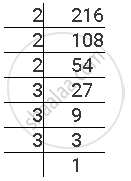Share

# The Following Numbers Are Not Perfect Cubes? 216 - CBSE Class 8 - Mathematics

ConceptCubes Some Interesting Patterns

#### Question

The following numbers are not perfect cubes?

216

#### Solution

The prime factorisation of 216 is as follows.216 = 2 × 2 × 2 × 3 × 3 × 3 = 23 × 33

Here, as each prime factor is appearing as many times as a perfect multiple of 3, therefore, 216 is a perfect cube

Is there an error in this question or solution?

#### APPEARS IN

NCERT Solution for Mathematics Textbook for Class 8 (2018 to Current)
Chapter 7: Cubes and Cube Roots
Ex. 7.10 | Q: 1.1 | Page no. 114
Solution The Following Numbers Are Not Perfect Cubes? 216 Concept: Cubes - Some Interesting Patterns.
S